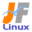Linux Kernel 2.6 Documentation: /usr/src/linux/Documentation/fb/vesafb.txt

# fb/vesafb.txt

vesafb \$B%U%l!<%`%P%C%U%!%G%P%\$%9%I%i%\$%P\$N@bL@(B [\$B%W%l%\$%s%F%-%9%HHG(B]
• \$B86Cx:n
• \$BK]Lu
• \$B%P!<%8%g%s(B: 2.6.12
• \$BK]LuF|;~(B: 2005/08/22
```

==================================
\$B\$3\$l\$O!"(B
linux-2.6.13-rc5/Documentation/fb/vesafb.txt \$B\$NOBLu(B
\$B\$G\$9!#(B
\$BK]LuCDBN!'(B JF \$B%W%m%8%'%/%H(B < http://www.linux.or.jp/JF/ >
\$BK]LuF|(B \$B!'(B 2005/8/8
\$B86Cx:npJs\$b4^\$a\$F(B)\$B\$K\$7!"(B
\$B%U%l!<%`%P%C%U%!%G%P%\$%9(B /dev/fb0 \$B\$H\$7\$F;HMQ\$7\$^\$9!#(B

This means we decide at boot time whenever we want to run in text or
graphics mode.  Switching mode later on (in protected mode) is
impossible; BIOS calls work in real mode only.  VESA BIOS Extensions
Version 2.0 are required, because we need a linear frame buffer.

\$B\$D\$^\$j\$3\$l\$O!"%F%-%9%H%b!<%I\$G.\$5\$/\$FFI\$_\$K\$/\$\$%U%)%s%H\$r;H\$o\$J\$/\$F\$b!"2wE,\$GBg\$-\$\$(B(\$B9b2rA|EY(B)\$B%3%s%=!<%k(B
(1024x768 \$B\$G(B 128\$B7e(B + 48\$B9T(B)\$B\$,;H\$(\$^\$9(B
* /dev/fb0 (=> \$B\$9\$Y\$F\$N!"(BVBE 2.0 \$B8_49%0%i%U%#%C%/%\!<%I\$G(B \$BHs%"%/%;%k(B X11\$B\$,;H\$(\$^\$9(B)
\$B>e\$G!"(BXF68_FBDev \$B\$r\$D\$+\$(\$^\$9!#(B
* \$B\$b\$C\$H\$b=EMW\$J(B: \$B%V!<%H%m%4(B :-)

\$BC;=j(B:

* graphic mode is slower than text mode...

* \$B%0%i%U%#%C%/%b!<%I\$O!"%F%-%9%H%b!<%I\$h\$jCY\$\$!%!%!%(B

How to use it?

\$B;H\$\$J}\$O!)(B
==============

Switching modes is done using the vga=... boot parameter.  Read
Documentation/svga.txt for details.

\$B%b!<%I@Z\$jBX\$(\$O!"%V!<%H%Q%i%a!<%?(B vga=... \$B\$G9T\$\$\$^\$9!#(B
\$B>\:Y\$O!"(BDocumentation/svga.txt \$B\$rFI\$s\$G\$/\$@\$5\$\$!#(B

You should compile in both vgacon (for text mode) and vesafb (for
graphics mode). Which of them takes over the console depends on
whenever the specified mode is text or graphics.

vgacon(\$B%F%-%9%H%b!<%IMQ(B) \$B\$H!"(Bvesafb(\$B%0%i%U%#%C%/%b!<%IMQ(B)\$B\$NN>J}\$r(B
\$B%3%s%Q%\$%k\$7\$J\$1\$l\$P\$\$\$1\$^\$;\$s!#(B
\$B\$I\$A\$i\$,%3%s%=!<%k\$K@\5/F0\$9\$k\$K\$O!"(B
\$BHV9f(B(mode-number) \$B\$r(B10\$B?J?t\$KJQ49\$7\$^\$9!#(B
2. \$BCm5-(B: LILO \$B\$N\$h\$j?7\$7\$\$HG\$G\$O!"HV9f\$NA0\$K(B 0x \$B\$r\$D\$1\$F\$"\$2\$l\$P!"(B
16\$B?J?t(B \$B\$GF0\$/\$h\$&\$K;W\$(\$k\$b\$N\$b\$"\$j\$^\$9!#(B

X11
===

XF68_FBDev should work just fine, but it is non-accelerated.  Running
another (accelerated) X-Server like XF86_SVGA might or might not work.
It depends on X-Server and graphics board.

XF68_FBDev \$B\$O!"\$&\$^\$/F0\$/\$O\$:\$G\$9!#\$7\$+\$7!"Hs%"%/%;%k\$K\$J\$j\$^\$9!#(B
\$BB>\$N(B XF86_SVGA \$B\$N\$h\$&\$J(B (\$B%"%/%;%k2=\$7\$?(B) \$B#X(B \$B%5!<%P\$O!"F0\$/\$+\$b\$7\$l\$J\$\$\$7!"F0\$+\$J\$\$\$+\$b\$7\$l\$^\$;\$s!#(B
X \$B%5!<%P\$H!"%0%i%U%#%C%/%\!<%I\$K0MB8\$7\$^\$9!#(B

The X-Server must restore the video mode correctly, else you end up

X \$B%5!<%P\$O@5\$7\$/%S%G%*%b!<%I\$rI|85\$7\$J\$1\$l\$P\$J\$j\$^\$;\$s!#(B
\$B\$G\$J\$1\$l\$P!"2u\$l\$?%3%s%=!<%k\$K\$J\$C\$F\$7\$^\$\$\$^\$9(B(vesafb \$B\$O\$3\$l\$K\$D\$\$\$F\$I\$&\$9\$k\$3\$H\$b\$G\$-\$^\$;\$s(B)\$B!#(B

Refresh rates

\$B%j%U%l%C%7%e%l!<%H(B
=============

There is no way to change the vesafb video mode and/or timings after
booting linux.  If you are not happy with the 60 Hz refresh rate, you
have these options:

Linux \$B\$,5/F0\$7\$?8e\$K!"(Bvesafb \$B%S%G%*%b!<%I\$*\$h\$S(B/\$B\$^\$?\$O!"%?%\$%_%s%0\$r@Z\$jBX\$(\$kl9g!"%S%G%*%b!<%I\$K1~\$8\$F!"(B
\$B%a%b%j\$r:FG[CV\$7\$^\$9!#(B
(Antonino Daplas \$B\$K\$h\$k!"(B2.5.66 patch/idea \$B\$O!">e=q\$-\$G\$-\$k\$h\$&\$K\$9\$k(B
(\$B%+!<%M%k\$O\$5\$i\$KB?\$/\$N(B fb \$B%a%b%j\$r3d\$jEv\$F\$k\$G\$7\$g\$&(B)\$B\$?\$a\$K!"(Btmb@iki.fi \$B\$K\$h\$j!"(B
2.4\$B7O\$K%P%C%/%]!<%H\$5\$l\$^\$7\$?(B)\$B!#(B

vtotal:n
if the video BIOS of your card incorrectly determines the total
amount of video RAM, use this option to override the BIOS (in MiB).

vtotal:n
\$B%+!<%I\$N%S%G%*(B BIOS \$B\$,%S%G%*(B RAM\$B\$NAmMFNL\$r@5\$7\$/G'<1\$G\$-\$J\$\$>l9g!"(B
\$B\$3\$N%*%W%7%g%s\$r;H\$\$!"(BBIOS (MiB \$BCf\$N(B) \$B\$r>e=q\$-\$7\$^\$9!#(B

Have fun!

\$B3Z\$7\$s\$G\$M!*(B

Gerd

--
Gerd Knorr <kraxel at goldbach dot in-berlin dot de>

Minor (mostly typo) changes
by Nico Schmoigl <schmoigl at rumms dot uni-mannheim dot de>
```